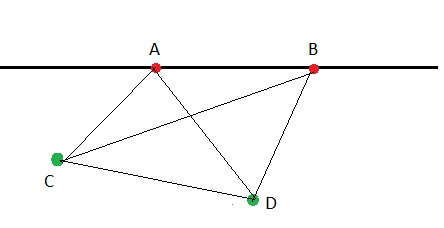# Count of triangles with total n points with m collinear in C++

We are given two variables n and m representing the number of points on a 2D plane. Out of n points, m points are collinear. The goal is to find the number of triangles that can be formed using these n points.

Collinear points − The points that lie on the same line are called collinear. Points A and B are collinear.Given n=4 (A,B,C,D ) , m=2 (A,B)

Number of triangles −

Choosing any three points out of 4 = 4C3

But collinear points cannot form triangle so remove possible triangles that will be counted above = 2C3

Total triangles= 4C3 - 2C3= 4-0 = 4 ( ABC, ACD, BCD, ABD )

For n and m = nC3 - mC3

Let us understand with examples.

Input − n=5, m=3

Output − Count of triangles with total n points with m collinear are − 9

Explanation − Total triangles = 5C3 - 3C3 = 10 - 1 = 9

Input − n=10, m=5

Output − Count of triangles with total n points with m collinear are − 110

Explanation − Total triangles = 10C3 - 5C3 = 120 - 10 = 110

## Approach used in the below program is as follows

We will create a pascal triangle for containing calculations of combinations. Every row is calculated using addition of previous row columns.

• Take variables n and m as input for a number of points.

• Function collinear_points(int n,int m) takes n and m and returns the count of triangles with total n points with m collinear.

• Set count = check(n, 3) - check(m, 3); ( for calculating nC3 - mC3 )

• Function check(int n, int r) takes n and r and returns value of nCr

• Take an array arr of length r+1.

• Set the whole array with 0’s using memset.

• Set arr = 1.

• Using two for loops from i=0 to i<=n. And j=min (i,r) to j>0 calculate the pascal triangle as arr[j]=arr[j]+arr[j-1].

• In the end we will get arr[r] as nCr. Return it.

• After the end of function check(). We will get count of triangles

• Return count as result.

## Example

Live Demo

#include <bits/stdc++.h>
using namespace std;
int check(int n, int r){
int arr[r+1];
memset(arr, 0, sizeof(arr));
arr = 1;
for (int i = 1; i <= n; i++){
for (int j = min(i, r); j > 0; j--){
arr[j] = arr[j] + arr[j-1];
}
}
return arr[r];
}
int collinear_points(int n,int m){
int count = check(n, 3) - check(m, 3);
return count;
}
int main(){
int n = 6, m = 2;
cout<<"Count of triangles with total n points with m collinear are: "<< collinear_points(n, m);
return 0;
}

## Output

If we run the above code it will generate the following output −

Count of triangles with total n points with m collinear are: 20